# 神经网络变得轻松（第十三部分）：批次常规化

### 内容

• 概述
• 1. 常规化的理论前提
• 2. 实现
• 2.1. 为我们的模型创建新的类
• 2.2. 前馈
• 2.3. 反馈
• 2.4. 神经网络基类的变化
• 3. 测试
• 结束语
• 参考
• 本文中用到的程序

### 1. 常规化的理论前提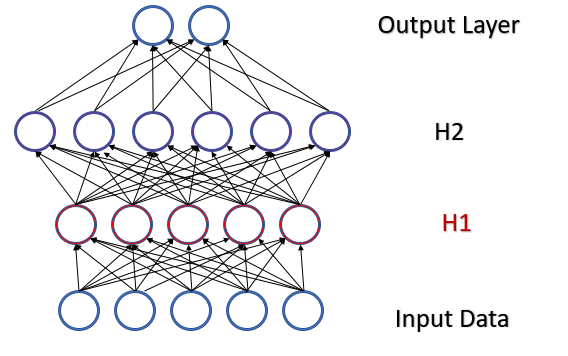2015 年 2 月，Sergey Ioffe 和 Christian Szegedy 提出了批次常规化作为内部协方差偏移问题的解决方案 。 该方法的思路是在特定时间间隔内把每个单独的神经元进行常规化，样本（批次）的中位数向零偏移，并令样本方差为 1。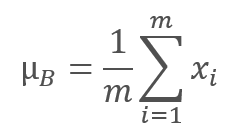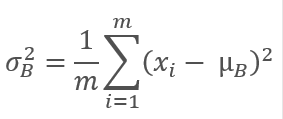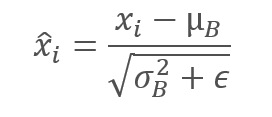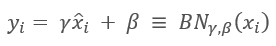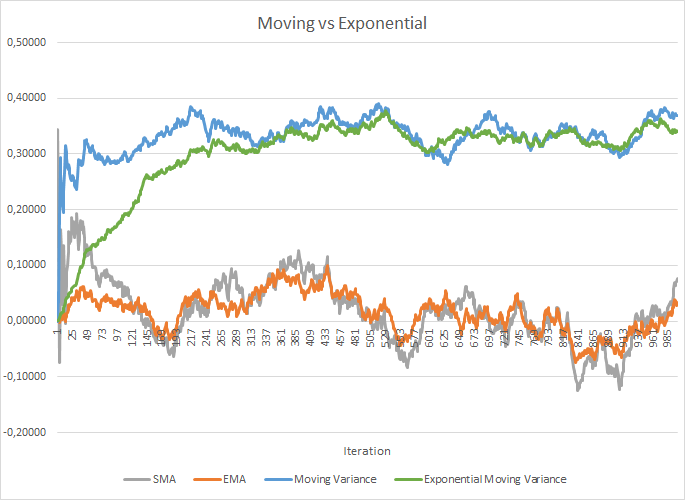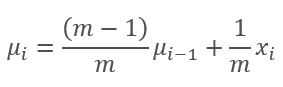,

• m 是批次大小,
• i 是迭代索引。

### 2. 实现

#### 2.1 为我们的模型创建新的类

```class CNeuronBatchNormOCL  :  public CNeuronBaseOCL
{
protected:
CNeuronBaseOCL    *PrevLayer;       ///< Pointer to the object of the previous layer
uint              iBatchSize;       ///< Batch size
CBufferDouble     *BatchOptions;    ///< Container of method parameters

///\ingroup neuron_base_ff
virtual bool      feedForward(CNeuronBaseOCL *NeuronOCL);               ///< \brief Feed Forward method of calling kernel ::BatchFeedForward().@param NeuronOCL Pointer to previous layer.

///\ingroup neuron_base_opt
virtual bool      updateInputWeights(CNeuronBaseOCL *NeuronOCL);        ///< Method for updating weights.\details Calling one of kernels ::UpdateBatchOptionsMomentum() or ::UpdateBatchOptionsAdam() in depends on optimization type (#ENUM_OPTIMIZATION).@param NeuronOCL Pointer to previous layer.

public:
/** Constructor */CNeuronBatchNormOCL(void);
/** Destructor */~CNeuronBatchNormOCL(void);
//---
virtual bool      Init(uint numOutputs, uint myIndex, COpenCLMy *open_cl, uint numNeurons, uint batchSize, ENUM_OPTIMIZATION optimization_type);
///< Method of initialization class.@param[in] numOutputs Number of connections to next layer.@param[in] myIndex Index of neuron in layer.@param[in] open_cl Pointer to #COpenCLMy object. #param[in] numNeurons Number of neurons in layer @param optimization_type Optimization type (#ENUM_OPTIMIZATION)@return Boolen result of operations.
//---
virtual int       getOutputIndex(void)          {  return (iBatchSize>1 ? Output.GetIndex() : PrevLayer.getOutputIndex());             }  ///< Get index of output buffer @return Index
//---
virtual int       getOutputVal(double &values[])   {  return (iBatchSize>1 ? Output.GetData(values) : PrevLayer.getOutputVal(values)); }  ///< Get values of output buffer @param[out] values Array of data @return number of items
virtual int       getOutputVal(CArrayDouble *values)   {  return (iBatchSize>1 ? Output.GetData(values) : PrevLayer.getOutputVal(values)); }  ///< Get values of output buffer @param[out] values Array of data @return number of items
virtual int       getGradient(double &values[])    {  return (iBatchSize>1 ? Gradient.GetData(values) : PrevLayer.getGradient(values));    }  ///< Get values of gradient buffer @param[out] values Array of data @return number of items
virtual CBufferDouble   *getOutput(void)           {  return (iBatchSize>1 ? Output : PrevLayer.getOutput());      }                 ///< Get pointer of output buffer @return Pointer to object
//---
virtual bool      calcInputGradients(CNeuronBaseOCL *NeuronOCL);          ///< Method to transfer gradient to previous layer by calling kernel ::CalcHiddenGradientBatch(). @param NeuronOCL Pointer to next layer.
//---
virtual bool      Save(int const file_handle);///< Save method @param[in] file_handle handle of file @return logical result of operation
virtual bool      Load(int const file_handle);///< Load method @param[in] file_handle handle of file @return logical result of operation
//---
virtual int       Type(void)        const                      {  return defNeuronBatchNormOCL;    }///< Identificator of class.@return Type of class
};
```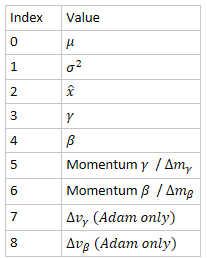```CNeuronBatchNormOCL::CNeuronBatchNormOCL(void)  :  iBatchSize(1)
{
PrevLayer=NULL;
BatchOptions=NULL;
}

```

```CNeuronBatchNormOCL::~CNeuronBatchNormOCL(void)
{
if(CheckPointer(PrevLayer)!=POINTER_INVALID)
PrevLayer=NULL;
if(CheckPointer(BatchOptions)!=POINTER_INVALID)
delete BatchOptions;
}

```

```bool CNeuronBatchNormOCL::Init(uint numOutputs,uint myIndex,COpenCLMy *open_cl,uint numNeurons,uint batchSize,ENUM_OPTIMIZATION optimization_type)
{
if(!CNeuronBaseOCL::Init(numOutputs,myIndex,open_cl,numNeurons,optimization_type))
return false;
activation=None;
iBatchSize=batchSize;
//---
if(CheckPointer(BatchOptions)!=POINTER_INVALID)
delete BatchOptions;
int count=(int)numNeurons*(optimization_type==SGD ? 7 : 9);
BatchOptions=new CBufferDouble();
if(CheckPointer(BatchOptions)==POINTER_INVALID || !BatchOptions.BufferInit(count,0))
return false;
//---
return true;
}

```

#### 2.2. 前馈

```__kernel void BatchFeedForward(__global double *inputs,
__global double *options,
__global double *output,
int batch
int optimization,
int activation)
{
if(batch<=1)
return;
int n=get_global_id(0);
int shift=n*(optimization==0 ? 7 : 9);
//---
for(int i=0;i<(optimization==0 ? 7 : 9);i++)
if(isnan(options[shift+i]))
options[shift+i]=0;
//---
double mean=(options[shift]*((double)batch-1)+inputs[n])/((double)batch);
double delt=inputs[n]-mean;
double variance=options[shift+1]*((double)batch-1.0)+pow(delt,2);
if(options[shift+1]>0)
variance/=(double)batch;
double nx=delt/sqrt(variance+1e-6);
//---
if(options[shift+3]==0)
options[shift+3]=1;
//---
double res=options[shift+3]*nx+options[shift+4];
switch(activation)
{
case 0:
res=tanh(clamp(res,-20.0,20.0));
break;
case 1:
res=1/(1+exp(-clamp(res,-20.0,20.0)));
break;
case 2:
if(res<0)
res*=0.01;
break;
default:
break;
}
//---
options[shift]=mean;
options[shift+1]=variance;
options[shift+2]=nx;
output[n]=res;
}

```

BatchFeedForward 内核构建算法相当简单，故我们可以继续创建从主程序调用内核的方法。 此功能将由 CNeuronBatchNormOCL::feedForward 方法实现。该方法的算法与其他类的相关方法类似。 该方法在参数中接收一个指向神经网络前一层的指针。

```bool CNeuronBatchNormOCL::feedForward(CNeuronBaseOCL *NeuronOCL)
{
if(CheckPointer(OpenCL)==POINTER_INVALID || CheckPointer(NeuronOCL)==POINTER_INVALID)
return false;
//---
PrevLayer=NeuronOCL;
if(iBatchSize<=1)
{
activation=(ENUM_ACTIVATION)NeuronOCL.Activation();
return true;
}
//---
if(CheckPointer(BatchOptions)==POINTER_INVALID)
{
int count=Neurons()*(optimization==SGD ? 7 : 9);
BatchOptions=new CBufferDouble();
if(!BatchOptions.BufferInit(count,0))
return false;
}
if(!BatchOptions.BufferCreate(OpenCL))
return false;
//---
uint global_work_offset= {0};
uint global_work_size;
global_work_size=Neurons();
if(!OpenCL.SetArgumentBuffer(def_k_BatchFeedForward,def_k_bff_inputs,NeuronOCL.getOutputIndex()))
return false;
if(!OpenCL.SetArgumentBuffer(def_k_BatchFeedForward,def_k_bff_options,BatchOptions.GetIndex()))
return false;
if(!OpenCL.SetArgumentBuffer(def_k_BatchFeedForward,def_k_bff_output,Output.GetIndex()))
return false;
if(!OpenCL.SetArgument(def_k_BatchFeedForward,def_k_bff_batch,iBatchSize))
return false;
if(!OpenCL.SetArgument(def_k_BatchFeedForward,def_k_bff_optimization,(int)optimization))
return false;
if(!OpenCL.SetArgument(def_k_BatchFeedForward,def_k_bff_activation,(int)activation))
return false;
ResetLastError();
//---
if(!OpenCL.Execute(def_k_BatchFeedForward,1,global_work_offset,global_work_size))
{
printf("Error of execution kernel Batch Feed Forward: %d",GetLastError());
return false;
}
return false;
BatchOptions.BufferFree();
//---
return true;
}

```

#### 2.3. 反馈

```__kernel void CalcHiddenGradientBatch(__global double *options,    ///<[in] Options matrix m*(7 or 9), where m - Number of neurons in previous layer
__global double *matrix_g,   ///<[in] Tensor of gradients at current layer
__global double *matrix_i,   ///<[in] Tensor of previous layer output
__global double *matrix_ig,  ///<[out] Tensor of gradients at previous layer
uint activation,             ///< Activation type (#ENUM_ACTIVATION)
int batch,                   ///< Batch size
int optimization             ///< Optimization type
)
{
if(batch<=1)
return;
//---
int n=get_global_id(0);
int shift=n*(optimization==0 ? 7 : 9);
//---
double inp=matrix_i[n];
double gnx=matrix_g[n]*options[shift+3];
double temp=1/sqrt(options[shift+1]+1e-6);
double gmu=(-temp)*gnx;
double gvar=(options[shift]*inp)/(2*pow(options[shift+1]+1.0e-6,3/2))*gnx;
double gx=temp*gnx+gmu/batch+gvar*2*inp/batch*pow((double)(batch-1)/batch,2.0);
//---
if(isnan(gx))
gx=0;
switch(activation)
{
case 0:
gx=clamp(gx+inp,-1.0,1.0)-inp;
gx=gx*(1-pow(inp==1 || inp==-1 ? 0.99999999 : inp,2));
break;
case 1:
gx=clamp(gx+inp,0.0,1.0)-inp;
gx=gx*(inp==0 || inp==1 ? 0.00000001 : (inp*(1-inp)));
break;
case 2:
if(inp<0)
gx*=0.01;
break;
default:
break;
}
}

```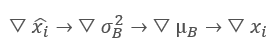```bool CNeuronBatchNormOCL::calcInputGradients(CNeuronBaseOCL *NeuronOCL)
{
if(CheckPointer(OpenCL)==POINTER_INVALID || CheckPointer(NeuronOCL)==POINTER_INVALID)
return false;
//---
if(iBatchSize<=1)
return (CheckPointer(PrevLayer)!=POINTER_INVALID);
//---
if(CheckPointer(BatchOptions)==POINTER_INVALID || !BatchOptions.BufferCreate(OpenCL))
return false;
//---
uint global_work_offset= {0};
uint global_work_size;
global_work_size=Neurons();
return false;
return false;
return false;
return false;
return false;
return false;
return false;
ResetLastError();
//---
{
printf("Error of execution kernel Batch CalcHiddenGradient: %d",GetLastError());
return false;
}
return false;
BatchOptions.BufferFree();
//---
return true;
}

```

```__kernel void UpdateBatchOptionsMomentum(__global double *options,     ///<[in,out] Options matrix m*7, where m - Number of neurons in previous layer
__global double *matrix_g,   ///<[in] Tensor of gradients at current layer
double learning_rates,       ///< Learning rates
double momentum              ///< Momentum multiplier
)
{
const int n=get_global_id(0);
const int shift=n*7;
//---
if(!isnan(delta.s0) && !isnan(delta.s1))
{
options[shift+5]=delta.s0;
options[shift+3]=clamp(options[shift+3]+delta.s0,-MAX_WEIGHT,MAX_WEIGHT);
options[shift+6]=delta.s1;
options[shift+4]=clamp(options[shift+4]+delta.s1,-MAX_WEIGHT,MAX_WEIGHT);
}
};

```

```__kernel void UpdateBatchOptionsAdam(__global double *options,     ///<[in,out] Options matrix m*9, where m - Number of neurons in previous layer
__global double *matrix_g,     ///<[in] Tensor of gradients at current layer
const double l,                ///< Learning rates
const double b1,               ///< First momentum multiplier
const double b2                ///< Second momentum multiplier
)
{
const int n=get_global_id(0);
const int shift=n*9;
//---
double2 delta=l*mt/sqrt(vt+1.0e-8);
if(isnan(delta.s0) || isnan(delta.s1))
return;
double2 weight=clamp((double2)(options[shift+3],options[shift+4])+delta,-MAX_WEIGHT,MAX_WEIGHT);
//---
if(!isnan(weight.s0) && !isnan(weight.s1))
{
options[shift+3]=weight.s0;
options[shift+4]=weight.s1;
options[shift+5]=mt.s0;
options[shift+6]=mt.s1;
options[shift+7]=vt.s0;
options[shift+8]=vt.s1;
}
};

```

```bool CNeuronBatchNormOCL::updateInputWeights(CNeuronBaseOCL *NeuronOCL)
{
if(CheckPointer(OpenCL)==POINTER_INVALID || CheckPointer(NeuronOCL)==POINTER_INVALID)
return false;
//---
if(iBatchSize<=1)
return (CheckPointer(PrevLayer)!=POINTER_INVALID);
//---
if(CheckPointer(BatchOptions)==POINTER_INVALID || !BatchOptions.BufferCreate(OpenCL))
return false;
uint global_work_offset= {0};
uint global_work_size;
global_work_size=Neurons();
//---
if(optimization==SGD)
{
if(!OpenCL.SetArgumentBuffer(def_k_UpdateBatchOptionsMomentum,def_k_buom_options,BatchOptions.GetIndex()))
return false;
return false;
if(!OpenCL.SetArgument(def_k_UpdateBatchOptionsMomentum,def_k_buom_learning_rates,eta))
return false;
if(!OpenCL.SetArgument(def_k_UpdateBatchOptionsMomentum,def_k_buom_momentum,alpha))
return false;
ResetLastError();
//---
if(!OpenCL.Execute(def_k_UpdateBatchOptionsMomentum,1,global_work_offset,global_work_size))
{
printf("Error of execution kernel UpdateBatchOptionsMomentum %d",GetLastError());
return false;
}
}
else
{
return false;
return false;
return false;
return false;
return false;
ResetLastError();
//---
{
printf("Error of execution kernel UpdateBatchOptionsAdam %d",GetLastError());
return false;
}
}
//---
return false;
BatchOptions.BufferFree();
//---
return true;
}

```

#### 2.4. 神经网络基类的变化

```#define defNeuronBatchNormOCL 0x7891      ///<Batchnorm neuron OpenCL \details Identified class #CNeuronBatchNormOCL

```

```#define def_k_BatchFeedForward         24 ///< Index of the kernel for Batch Normalization Feed Forward process (#CNeuronBathcNormOCL)
#define def_k_bff_inputs               0  ///< Inputs data tensor
#define def_k_bff_options              1  ///< Tensor of variables
#define def_k_bff_output               2  ///< Tensor of output data
#define def_k_bff_batch                3  ///< Batch size
#define def_k_bff_optimization         4  ///< Optimization type
#define def_k_bff_activation           5  ///< Activation type
//---
#define def_k_CalcHiddenGradientBatch  25 ///< Index of the Kernel of the Batch neuron to transfer gradient to previous layer (#CNeuronBatchNormOCL)
#define def_k_bchg_options             0  ///<[in] Options matrix m*(7 or 9), where m - Number of neurons in previous layer
#define def_k_bchg_matrix_g            1  ///<[in] Tensor of gradients at current layer
#define def_k_bchg_matrix_i            2  ///<[in] Tensor of previous layer output
#define def_k_bchg_matrix_ig           3  ///<[out] Tensor of gradients at previous layer
#define def_k_bchg_activation          4  ///< Activation type (#ENUM_ACTIVATION)
#define def_k_bchg_batch               5  ///< Batch size
#define def_k_bchg_optimization        6  ///< Optimization type
//---
#define def_k_UpdateBatchOptionsMomentum  26 ///< Index of the kernel for Describe the process of SGD optimization options for the Batch normalization Neuron (#CNeuronBatchNormOCL).
#define def_k_buom_options                0  ///<[in] Options matrix m*(7 or 9), where m - Number of neurons in previous layer
#define def_k_buom_matrix_g               1  ///<[in] Tensor of gradients at current layer
#define def_k_buom_learning_rates         2  ///< Learning rates
#define def_k_buom_momentum               3  ///< Momentum multiplier
//---
#define def_k_UpdateBatchOptionsAdam      27 ///< Index of the kernel for Describe the process of Adam optimization options for the Batch normalization Neuron (#CNeuronBatchNormOCL).
#define def_k_buoa_options                0  ///<[in] Options matrix m*(7 or 9), where m - Number of neurons in previous layer
#define def_k_buoa_matrix_g               1  ///<[in] Tensor of gradients at current layer
#define def_k_buoa_l                      2  ///< Learning rates
#define def_k_buoa_b1                     3  ///< First momentum multiplier
#define def_k_buoa_b2                     4  ///< Second momentum multiplier

```

```CNet::CNet(CArrayObj *Description)
{
if(CheckPointer(Description)==POINTER_INVALID)
return;
//---
................
................
................
//---
for(int i=0; i<total; i++)
{
prev=desc;
desc=Description.At(i);
if((i+1)<total)
{
next=Description.At(i+1);
if(CheckPointer(next)==POINTER_INVALID)
return;
}
else
next=NULL;
int outputs=(next==NULL || (next.type!=defNeuron && next.type!=defNeuronBaseOCL) ? 0 : next.count);
temp=new CLayer(outputs);
int neurons=(desc.count+(desc.type==defNeuron || desc.type==defNeuronBaseOCL ? 1 : 0));
if(CheckPointer(opencl)!=POINTER_INVALID)
{
................
................
................
CNeuronBatchNormOCL *batch=NULL;
switch(desc.type)
{
................
................
................
................
//---
case defNeuronBatchNormOCL:
batch=new CNeuronBatchNormOCL();
if(CheckPointer(batch)==POINTER_INVALID)
{
delete temp;
return;
}
if(!batch.Init(outputs,0,opencl,desc.count,desc.window,desc.optimization))
{
delete batch;
delete temp;
return;
}
batch.SetActivationFunction(desc.activation);
{
delete batch;
delete temp;
return;
}
batch=NULL;
break;
//---
default:
return;
break;
}
}
................
................
................
................
//---
if(CheckPointer(opencl)==POINTER_INVALID)
return;
//--- create kernels
opencl.SetKernelsCount(28);
................
................
................
................
opencl.KernelCreate(def_k_BatchFeedForward,"BatchFeedForward");
opencl.KernelCreate(def_k_UpdateBatchOptionsMomentum,"UpdateBatchOptionsMomentum");
//---
return;
}

```

```bool CNet::Load(string file_name,double &error,double &undefine,double &forecast,datetime &time,bool common=true)
{
if(MQLInfoInteger(MQL_OPTIMIZATION) || MQLInfoInteger(MQL_TESTER) || MQLInfoInteger(MQL_FORWARD) || MQLInfoInteger(MQL_OPTIMIZATION))
return false;
//---
................
................
................
//---
if(CheckPointer(opencl)==POINTER_INVALID)
{
opencl=new COpenCLMy();
if(CheckPointer(opencl)!=POINTER_INVALID && !opencl.Initialize(cl_program,true))
delete opencl;
else
{
//--- create kernels
opencl.SetKernelsCount(28);
................
................
................
opencl.KernelCreate(def_k_BatchFeedForward,"BatchFeedForward");
opencl.KernelCreate(def_k_UpdateBatchOptionsMomentum,"UpdateBatchOptionsMomentum");
}
}
................
................
................
................
................
}

```

```bool CLayer::Load(const int file_handle)
{
iFileHandle=file_handle;
return false;
if(CheckPointer(m_data)==POINTER_INVALID)
return false;
//---
CNeuronBaseOCL *ocl=NULL;
CNeuronBase    *cpu=NULL;
switch(m_data.Type())
{
case defNeuronBaseOCL:
case defNeuronConvOCL:
case defNeuronAttentionOCL:
case defNeuronMHAttentionOCL:
case defNeuronMLMHAttentionOCL:
case defNeuronDropoutOCL:
case defNeuronBatchNormOCL:
ocl=m_data;
iOutputs=ocl.getConnections();
break;
default:
cpu=m_data;
iOutputs=cpu.getConnections().Total();
break;
}
//---
return true;
}

```

```bool CNeuronBaseOCL::FeedForward(CObject *SourceObject)
{
if(CheckPointer(SourceObject)==POINTER_INVALID)
return false;
//---
CNeuronBaseOCL *temp=NULL;
switch(SourceObject.Type())
{
case defNeuronBaseOCL:
case defNeuronConvOCL:
case defNeuronAttentionOCL:
case defNeuronMHAttentionOCL:
case defNeuronMLMHAttentionOCL:
case defNeuronDropoutOCL:
case defNeuronBatchNormOCL:
temp=SourceObject;
return feedForward(temp);
break;
}
//---
return false;
}

{
if(CheckPointer(TargetObject)==POINTER_INVALID)
return false;
//---
................
................
................
CNeuronBatchNormOCL *batch=NULL;
switch(TargetObject.Type())
{
................
................
................
case defNeuronBatchNormOCL:
batch=TargetObject;
temp=GetPointer(this);
break;
}
//---
return false;
}

bool CNeuronBaseOCL::UpdateInputWeights(CObject *SourceObject)
{
if(CheckPointer(SourceObject)==POINTER_INVALID)
return false;
//---
CNeuronBaseOCL *temp=NULL;
switch(SourceObject.Type())
{
case defNeuronBaseOCL:
case defNeuronConvOCL:
case defNeuronAttentionOCL:
case defNeuronMHAttentionOCL:
case defNeuronMLMHAttentionOCL:
case defNeuronDropoutOCL:
case defNeuronBatchNormOCL:
temp=SourceObject;
return updateInputWeights(temp);
break;
}
//---
return false;
}

```

### 3. 测试

```      //--- 0
CLayerDescription *desc=new CLayerDescription();
if(CheckPointer(desc)==POINTER_INVALID)
return INIT_FAILED;
desc.count=(int)HistoryBars*12;
desc.type=defNeuronBaseOCL;
desc.activation=None;
return INIT_FAILED;
//--- 1
desc=new CLayerDescription();
if(CheckPointer(desc)==POINTER_INVALID)
return INIT_FAILED;
desc.count=(int)HistoryBars*12;
desc.type=defNeuronBatchNormOCL;
desc.window=100;
desc.activation=TANH;
return INIT_FAILED;
//--- 2
desc=new CLayerDescription();
if(CheckPointer(desc)==POINTER_INVALID)
return INIT_FAILED;
desc.count=(int)HistoryBars;
desc.type=defNeuronConvOCL;
desc.window=12;
desc.step=12;
desc.window_out=24;
desc.activation=None;
return INIT_FAILED;
//--- 3
desc=new CLayerDescription();
if(CheckPointer(desc)==POINTER_INVALID)
return INIT_FAILED;
desc.count=(int)HistoryBars*24;
desc.type=defNeuronBatchNormOCL;
desc.window=100;
desc.activation=SIGMOID;
return INIT_FAILED;
//--- 4
desc=new CLayerDescription();
if(CheckPointer(desc)==POINTER_INVALID)
return INIT_FAILED;
desc.count=(int)HistoryBars;
desc.type=defNeuronMLMHAttentionOCL;
desc.window=24;
desc.window_out=4;
desc.layers=5;
desc.activation=SIGMOID;
return INIT_FAILED;
//--- 5
desc=new CLayerDescription();
if(CheckPointer(desc)==POINTER_INVALID)
return INIT_FAILED;
desc.count=200;
desc.type=defNeuron;
desc.activation=None;
return INIT_FAILED;
//--- 6
desc=new CLayerDescription();
if(CheckPointer(desc)==POINTER_INVALID)
return INIT_FAILED;
desc.count=200;
desc.type=defNeuronBatchNormOCL;
desc.window=100;
desc.activation=TANH;
return INIT_FAILED;
//--- 7
desc=new CLayerDescription();
if(CheckPointer(desc)==POINTER_INVALID)
return INIT_FAILED;
desc.count=200;
desc.type=defNeuron;
desc.activation=TANH;
return INIT_FAILED;
//--- 8
desc=new CLayerDescription();
if(CheckPointer(desc)==POINTER_INVALID)
return INIT_FAILED;
desc.count=3;
desc.type=defNeuron;
desc.activation=SIGMOID;

```

EA 基于 EURUSD，H1 时间帧进行了测试。 与之前的测试类似，将 20 根最新烛条的数据输入到神经网络之中。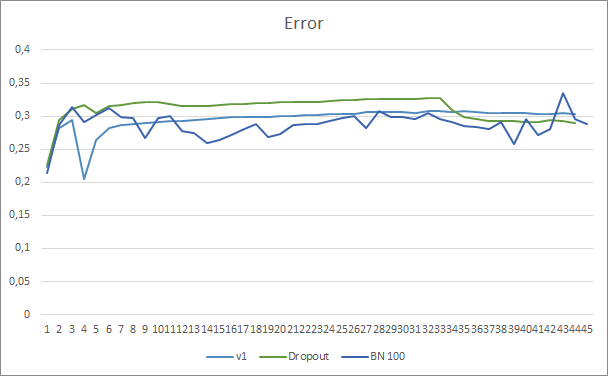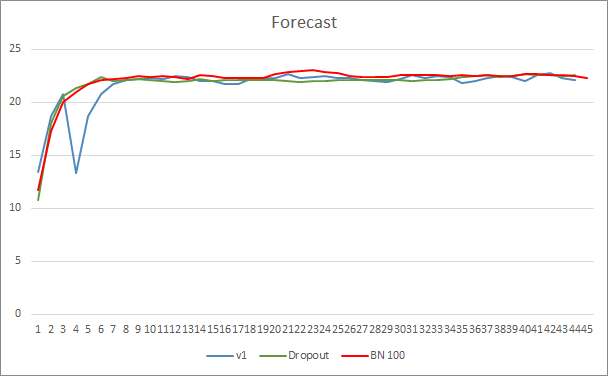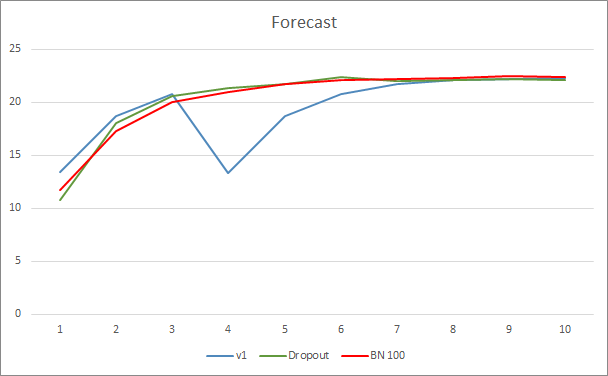### 参考

1. 神经网络变得轻松
2. 神经网络变得轻松（第二部分）：网络训练和测试
3. 神经网络变得轻松（第三部分）：卷积网络
4. 神经网络变得轻松（第四部分）：循环网络
5. 神经网络变得轻松（第五部分）：OpenCL 中的多线程计算
6. 神经网络变得轻松（第六部分）：神经网络学习率实验
7. 神经网络变得轻松（第七部分）：自适应优化方法
8. 神经网络变得轻松（第八部分）：关注机制
9. 神经网络变得轻松（第九部分）：操作归档
10. 神经网络变得轻松（第十部分）：多目击者关注
11. 神经网络变得轻松（第十一部分）：自 GPT 获取
12. 神经网络变得轻松（第十二部分）：舍弃
13. 批次常规化：通过减少内部协变量偏移来加速深度网络训练
14. 层常规化

### 本文中用到的程序

# 发行 类型 说明
1 Fractal_OCL_AttentionMLMH_b.mq5 智能交易系统   一款含有分类神经网络（输出层有 3 个神经元）的智能交易系统，采用 GTP 架构，有 5 个关注层 + BatchNorm
2 NeuroNet.mqh 类库 用于创建神经网络的类库
3 NeuroNet.cl 代码库 OpenCL 程序代码库
4 NN.chm HTML 帮助 一个编译后的函数库帮助 CHM 文件。

## 量化课程

移动端课程